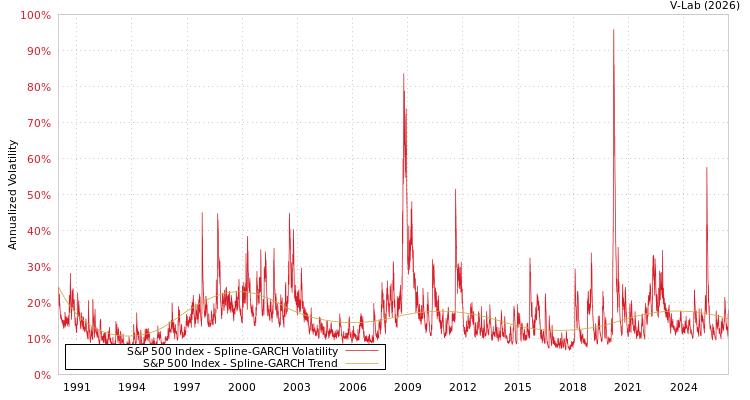##### S&P 500 Index Spline-GARCH Volatility Analysis
###### Volatility Prediction for Monday, October 25th, 2021:12.37% (-0.56%)

Analysis last updated: Friday, October 22, 2021, 09:47 PM UTC

###### Date Range:

from

to

6M ·

1Y ·

2Y ·

5Y ·

10Y ·

Allparamt-stat
$\omega$1.08157.03
$\alpha$0.10209.04
$\beta$0.854758.94
${\gamma }_{1}$-0.0032-0.06
${\gamma }_{2}$0.10891.38
${\gamma }_{3}$-0.1822-3.37
${\gamma }_{4}$0.02610.50
${\gamma }_{5}$0.17593.51
${\gamma }_{6}$-0.2448-5.46
${\gamma }_{7}$0.16233.01
${\gamma }_{8}$-0.0194-0.27
${\gamma }_{9}$-0.0343-0.31
Estimation Period:
Jan 1, 1990 to Oct 22, 2021